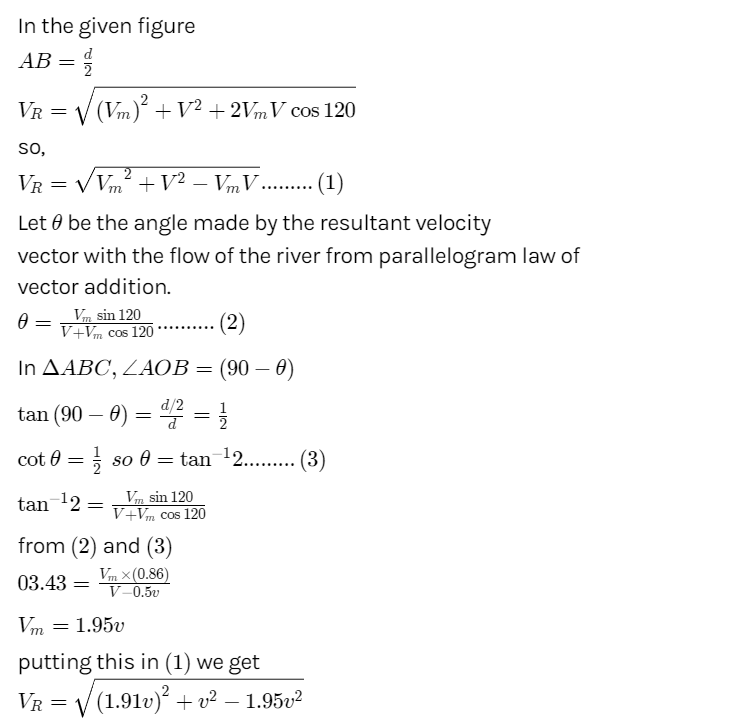#### Thank you for registering.

One of our academic counsellors will contact you within 1 working day.

Click to Chat

1800-5470-145

+91 7353221155

CART 0

• 0
MY CART (5)

Use Coupon: CART20 and get 20% off on all online Study Material

ITEM
DETAILS
MRP
DISCOUNT
FINAL PRICE
Total Price: Rs.

There are no items in this cart.
Continue Shopping

# A swimmer crosses a river of wifth d flowing at velocity v. While swimming he keeps himself always at an angle of 120degree with river and on reaching the other end he finds a drift of d/2 in the direction of flow of river .Find the speed of the swimmer with respect to the river.  Search ResultsWeb resultsRituraj Tiwari
11 months ago16 days ago

AB =  d / 2

V R  = √ (V  m  )^2  +V^2  + 2Vm Vcos120

V R  =   √V  m^ 2  +V^2  −V  m  V     .......(1)

• Let θ be the angle made by the resultant velocity  vector.
• From parallelogram law of vector addition.

θ = V mcos(120) /  V+  V m sin(120)   .......(2)

In ΔABC, ∠AOB = (90−θ)

tan(90−θ) =  d/2 ÷ d   =  1/2

​cotθ =   1/2 so θ = tan^−1  2  .......(3)

tan^−1  2 = V mcos(120) /  V+  V m sin(120)

From equations (2) and (3)

03.43 = Vm x 0.86 / V - 0.5 v

Vm = 1.95 v

Putting this in equation (1) we get.

V R  = √ (1.91v)^2 + v^2 + - 1.95 v^2

Hence the speed of the swimmer with respect to river is V R  = √ (1.91v)^2 + v^2 + - 1.95 v^2